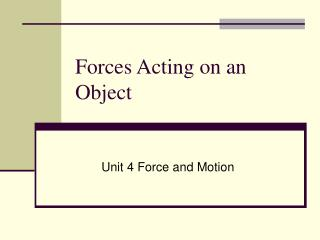# Forces Acting on an Object - PowerPoint PPT PresentationDownload PresentationForces Acting on an Object

Forces Acting on an Object
Download Presentation## Forces Acting on an Object

- - - - - - - - - - - - - - - - - - - - - - - - - - - E N D - - - - - - - - - - - - - - - - - - - - - - - - - - -
##### Presentation Transcript

1. Forces Acting on an Object Unit 4 Force and Motion

2. Applied ForceFapp • An applied force is a force which is applied to an object by a person or another object. If a person is pushing a desk across the room, then there is an applied force acting upon the object. The applied force is the force exerted on the desk by the person.

3. Gravity Force(also known as Weight)Fgrav • The force of gravity is the force with which the earth, moon, or other massively large object attracts another object towards itself. By definition, this is the weight of the object. All objects upon earth experience a force of gravity which is directed "downward" towards the center of the earth. The force of gravity on earth is always equal to the weight of the object as found by the equation: • Fgrav = m * g • where g = 9.8 m/s2 (on Earth) and m = mass (in kg) • (Caution: do not confuse weight with mass.)

4. Normal ForceFnorm • The normal force is the support force exerted upon an object which is in contact with another stable object. For example, if a book is resting upon a surface, then the surface is exerting an upward force upon the book in order to support the weight of the book. On occasions, a normal force is exerted horizontally between two objects which are in contact with each other. For instance, if a person leans against a wall, the wall pushes horizontally on the person.

5. Friction ForceFfrict • The friction force is the force exerted by a surface as an object moves across it or makes an effort to move across it. There are at least two types of friction force - sliding and static friction. Thought it is not always the cast, the friction force often opposes the motion of an object. For example, if a book slides across the surface of a desk, then the desk exerts a friction force in the opposite direction of its motion. Friction results from the two surfaces being pressed together closely, causing intermolecular attractive forces between molecules of different surfaces. As such, friction depends upon the nature of the two surfaces and upon the degree to which they are pressed together. The maximum amount of friction force which a surface can exert upon an object can be calculated using the formula below:

6. Air Resistance ForceFair • The air resistance is a special type of frictional force which acts upon objects as they travel through the air. The force of air resistance is often observed to oppose the motion of an object. This force will frequently be neglected due to its negligible magnitude (and due to the fact that it is mathematically difficult to predict its value). It is most noticeable for objects which travel at high speeds (e.g., a skydiver or a downhill skier) or for objects with large surface areas.

7. Tension ForceFtens • The tension force is the force which is transmitted through a string, rope, cable or wire when it is pulled tight by forces acting from opposite ends. The tension force is directed along the length of the wire and pulls equally on the objects on the opposite ends of the wire.

8. Spring ForceFspring • The spring force is the force exerted by a compressed or stretched spring upon any object which is attached to it. An object which compresses or stretches a spring is always acted upon by a force which restores the object to its rest or equilibrium position. For most springs (specifically, for those which are said to obey "Hooke's Law"), the magnitude of the force is directly proportional to the amount of stretch or compression of the spring.